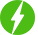# Complete Data Science BootCamp

84

Complete Data Science BootCamp, Learn about Data Science, Machine Learning, and Deep Learning, and build 5 different projects.

## Course Description

Data science is the field that encompasses the various techniques and methods used to extract insights and knowledge from data. Machine learning (ML) and deep learning (DL) are both subsets of data science, and they are often used together to analyze and understand data.

In data science, ML algorithms are often used to build predictive models that can make predictions based on historical data. These models can be used for tasks such as classification, regression, and clustering. ML algorithms include linear regression, decision trees, and k-means.

DL, on the other hand, is a subset of ML that is based on artificial neural networks with multiple layers, which allows the system to learn and improve through experience. DL is particularly well-suited for tasks such as image recognition, speech recognition, and natural language processing. DL algorithms include convolutional neural networks (CNNs) and recurrent neural networks (RNNs).

In a data science project, DL models are often used in combination with other techniques such as feature engineering, data cleaning, and visualization, to extract insights and knowledge from data. For instance, DL models can be used to automatically extract features from images, and then these features can be used in a traditional ML model.

In summary, Data science is the field that encompasses various techniques and methods to extract insights and knowledge from data, ML and DL are subsets of data science that are used to analyze and understand data, ML is used to build predictive models and DL is used to model complex patterns and relationships in data. Both ML and DL are often used together in data science projects to extract insights and knowledge from data.

IN THIS COURSE YOU WILL LEARN ABOUT :

• Life Cycle of a Data Science Project.
• Python libraries like Pandas and Numpy are used extensively in Data Science.
• Matplotlib and Seaborn for Data Visualization.
• Data Preprocessing steps like Feature Encoding, Feature Scaling, etc…
• Machine Learning Fundamentals and different algorithms
• Cloud Computing for Machine Learning
• Deep Learning
• 5 projects like Diabetes Prediction, Stock Price Prediction, etc…

Free \$84.99
We will be happy to hear your thoughtsRegister New Account
• Total (0)
0